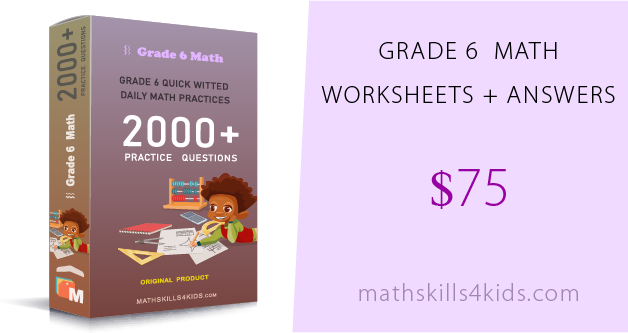You will find worksheets for every skill level, from easy to challenging, and with answers included.

### Samples of 6th Grade Printable Math Worksheets Pdf

•

Our sixth-grade math worksheets with answers are designed to help your kids practice and review their 6th-grade math skills and prepare for tests and quizzes that require knowledge of fractions, decimals, ratios, integers, expressions, equations, coordinate planes, area, perimeter, volume, surface area, statistics, probability, etc.

These 6th Grade Printable Math Worksheets Pdf with Answers and Explanations align with the common core standards and thoroughly review the essential skills and concepts for 6th-grade math. There are fun and engaging. They also have colorful graphics, word problems, exciting exercises, and challenges to motivate your students. The content also has answer keys showing detailed steps and explanations for each problem. This way, your kids can check their work and learn from their mistakes.

Let's look at some of the worksheets you can get from this fabulous collection!

•• ### Grade 6 whole numbers worksheets

Whole numbers worksheets will help your kids review the place value of digits in whole numbers up to millions and how to write numbers in words and Roman numerals. It also has some problems in finding the greatest common factor and the least common multiple of two or more numbers. This is a great way to reinforce the basics of number sense and operations.

• ### Grade 6 multiplication worksheets

Multiplication worksheets will challenge your kids to multiply three or more numbers using different strategies, such as grouping, distributive property, and area models. It also has some word problems that require them to apply their multiplication skills in real-life situations. This is a great way to develop their problem-solving and reasoning skills.

• ### Grade 6 division worksheets

Division worksheets will help your kids practice dividing whole numbers by two-digit and three-digit divisors, as well as decimals by whole numbers. It also has some problems with estimating quotients and interpreting remainders. This is a great way to enhance their accuracy and fluency in the division.

• ### Grade 6 exponents and square roots worksheets

Exponents and square roots worksheets will help your kids learn how to write multiplication expressions using exponents and convert them between standard form and scientific notation. The worksheets also include problems in finding the square root of perfect squares and estimating the square root of non-perfect squares. This is a great way to introduce them to powers and roots.

• ### Grade 6 number theory worksheets

Number theory worksheets will help your kids learn how to write the prime factorization of numbers using exponents and identify prime and composite numbers. It also has some problems in finding the greatest common factor and the least common multiple of two or more numbers using prime factorization. This is a great way to deepen their understanding of number properties and relationships.

• ### Grade 6 decimals worksheets

Decimals worksheets will help your kids review how to represent decimals on a number line and compare and order decimals. It also has some problems with adding, subtracting, multiplying, and dividing decimals using various methods. This is a great way to reinforce their skills in decimal operations.

• ### Grade 6 fractions and mixed numbers worksheets

Fractions and mixed numbers worksheets will help your kids review how to represent fractions on a number line and compare and order fractions with like and unlike denominators. It also has some problems with adding, subtracting, multiplying, and dividing fractions and mixed numbers using various models and strategies. This is a great way to enhance their skills in fraction operations.

• ### Grade 6 ratios worksheets

Ratios worksheets will help your kids compare quantities using ratios and rates and solve problems involving proportions and unit rates.

• ### Grade 6 integers worksheets

Integers worksheets will help your kids to learn how to represent, compare, order, add, subtract, multiply, and divide positive and negative integers using various models and methods.

• ### Grade 6 expressions and equations worksheets

Expressions and equations worksheets will help your kids to learn how to write, simplify, evaluate, and solve algebraic expressions and equations using properties of operations and inverse operations.

• ### Grade 6 coordinate planes worksheets

Coordinate planes worksheets will help to plot points, identify quadrants, find distances, and graph linear equations on the coordinate plane.

• ### Grade 6 area, perimeter, volume, and surface area worksheets

Area, perimeter, volume, and surface area worksheets will help your kids to learn how to find the area and perimeter of polygons, the volume and surface area of prisms and pyramids, and how to apply the formulas to real-world situations.

• ### Grade 6 statistics and probability worksheets

Statistics and probability worksheets will help your kids to learn how to organize data using dot plots, histograms, box plots, mean, median, quartiles, interquartile range, and outliers, and how to calculate simple probabilities using fractions, decimals, percents, and ratios.